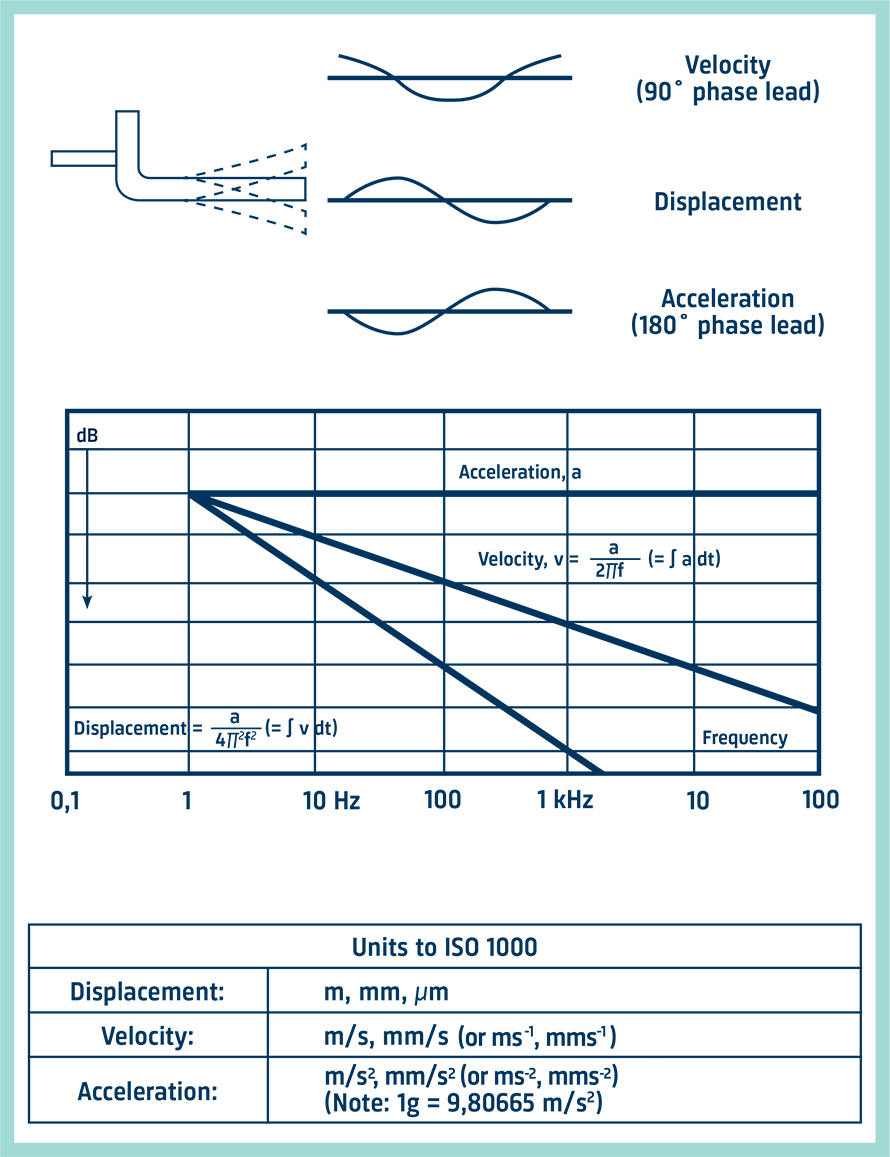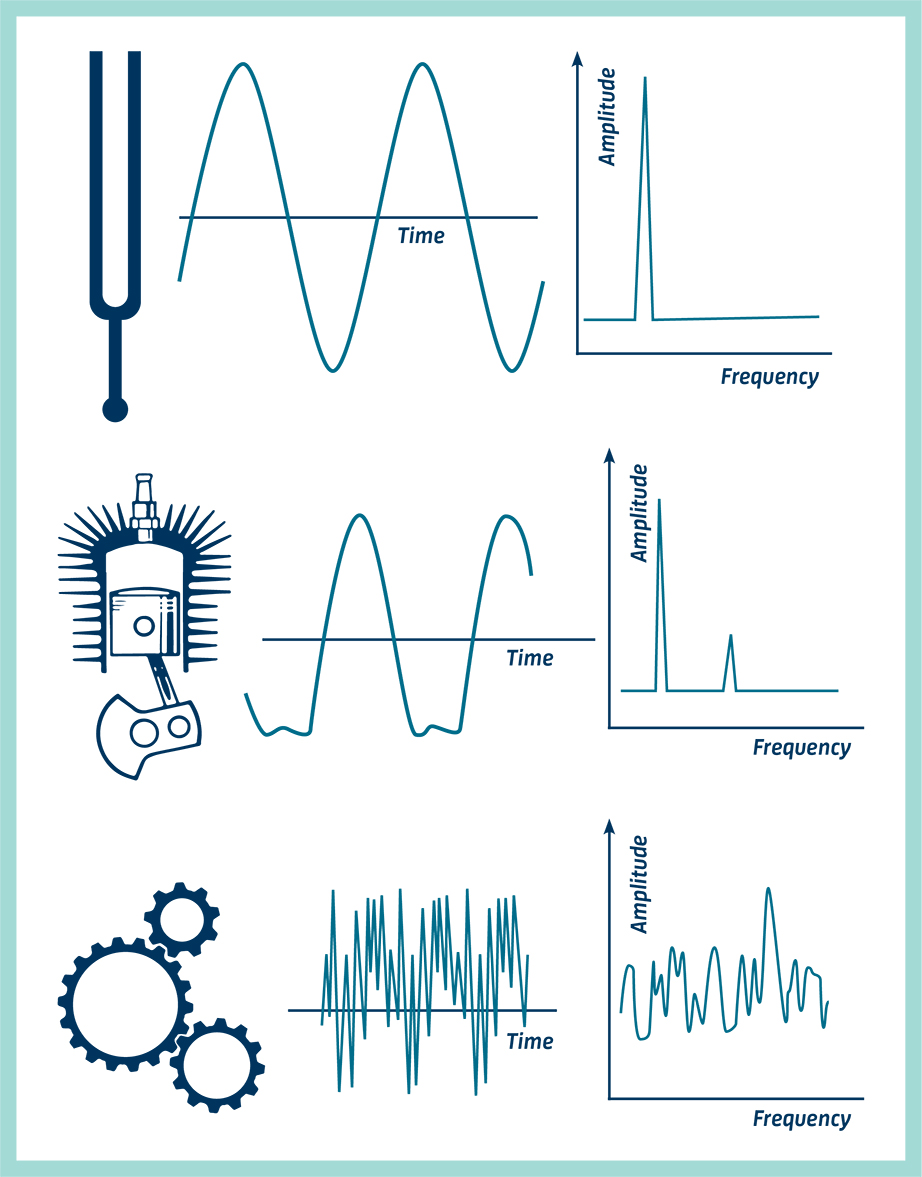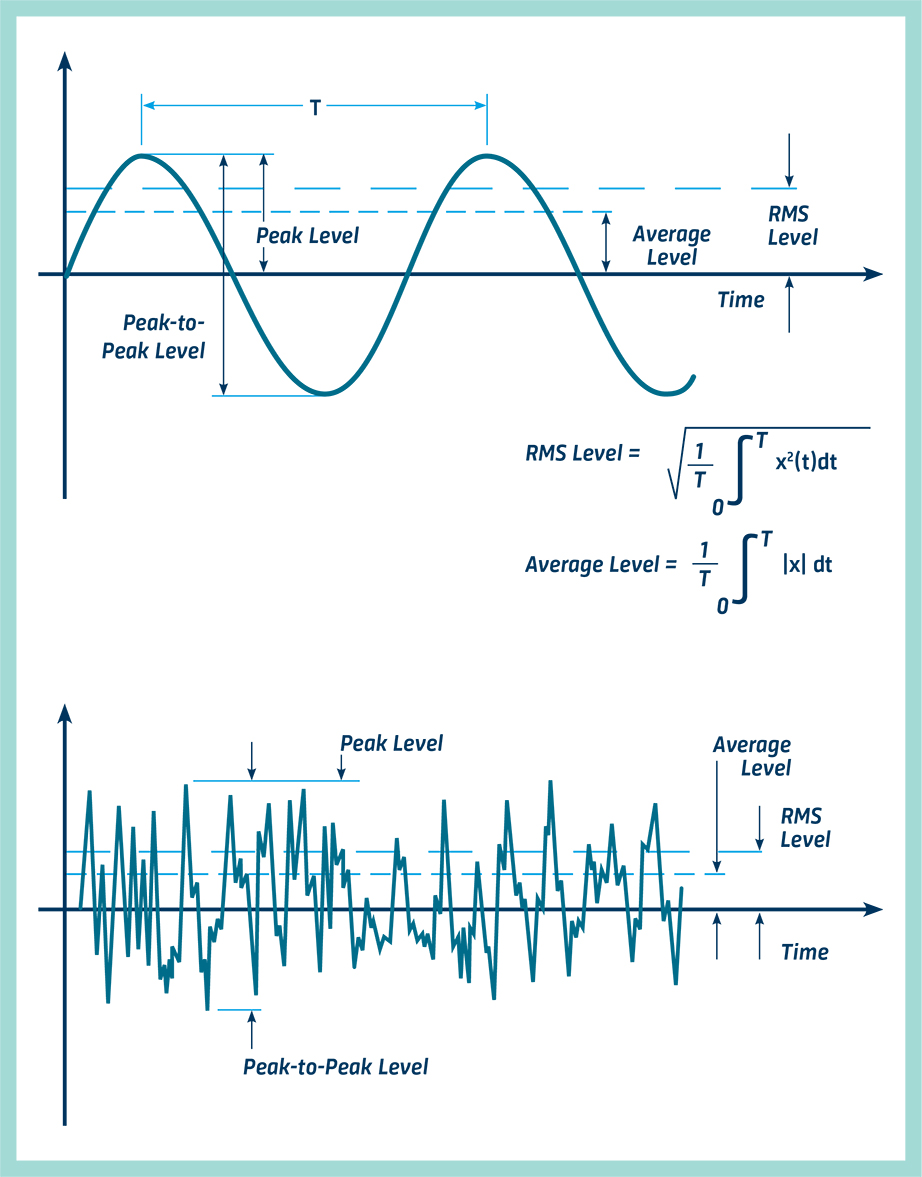# Vibration Measurement: The Complete Guide

As vibration isolation and reduction techniques have become an integral part of machine design, the need for accurate measurement and analysis of mechanical vibration has grown. Using accelerometers to convert vibratory motion into an electrical signal, the process of vibration measurement and analysis is ably performed by the versatile abilities of modern electronics.CONTENTS

GET THE FULL HANDBOOK
VIBRATION MEASUREMENT
BY BRÜEL & KJÆR

## What Is Vibration?

A body is said to vibrate when it describes an oscillating motion about a reference position. The number of times a complete motion cycle takes place during the period of a second is called the frequency and is measured in hertz (Hz).

The motion can consist of a single component occurring at a single frequency, as with a tuning fork, or of several components occurring at different frequencies simultaneously, for example, with the piston motion of an internal combustion engine.

Vibration signals in practice usually consist of very many frequencies occurring simultaneously so that we cannot immediately see just by looking at the amplitude-time pattern, how many components there are, and at what frequencies they occur. These components can be revealed by plotting vibration amplitude against frequency. The breaking down of vibration signals into individual frequency components is called frequency analysis, a technique which may be considered the cornerstone of diagnostic vibration measurements.

The graph showing the vibration level as a function of frequency is called a frequency spectrogram.

When frequency analyzing machine vibrations, we normally find several prominent periodic frequency components that are directly related to the fundamental movements of various parts of the machine. With frequency analysis, we are therefore able to track down the source of undesirable vibration.

## Where do Vibrations Come From?

In practice, it is very difficult to avoid vibration. It usually occurs because of the dynamic effects of manufacturing tolerances, clearances, rolling and rubbing contact between machine parts, and out-of-balance forces in rotating and reciprocating members. Often, small insignificant vibrations can excite the resonant frequencies of some other structural parts and be amplified into major vibration and noise sources.VIBRATION MEASUREMENT

Sometimes though, mechanical vibration performs a useful job. For example, we generate vibration intentionally in component feeders, concrete compactors, ultrasonic cleaning baths, rock drills, and pile drivers. Vibration testing machines are used extensively to impart a controlled level of vibration energy to products and sub-assemblies where it is required to examine their physical or functional response and ascertain their resistibility to vibration environments.

A fundamental requirement in all vibration work, whether it is in the design of machines that utilize its energies or in the creation and maintenance of smoothly running mechanical products, is the ability to obtain an accurate description of the vibration by measurement and analysis.

## Quantifying the Vibration Level

The vibration amplitude, which is the characteristic that describes the severity of the vibration, can be quantified in several ways. On the diagram, the relationship between the peak-to-peak level, the peak level, the average level, and the RMS level of a sinewave is shown.

The peak-to-peak value is valuable in that it indicates the maximum excursion of the wave, a useful quantity where, for example, the vibratory displacement of a machine part is critical for maximum stress or mechanical clearance considerations.

The peak value is particularly valuable for indicating the level of short duration shocks etc. But, as can be seen from the drawing, peak values only indicate what maximum level has occurred, no account is taken of the time history of the wave.

The rectified average value, on the other hand, does take the time history of the wave into account but is considered of limited practical interest because it has no direct relationship with any useful physical quantity.

The RMS value is the most relevant measure of amplitude because it both takes the time history of the wave into account and gives an amplitude value that is directly related to the energy content, and therefore the destructive abilities of the vibration.## Acceleration, Velocity, and Displacement

#### Measuring Units

When we looked at the vibrating tuning fork, we considered the amplitude of the wave as the physical displacement of the fork ends to either side of the rest position. In addition to Displacement, we can also describe the movement of the fork leg in terms of its velocity and its acceleration. The form and period of the vibration remain the same whether it is the displacement, velocity, or acceleration that is being considered. The main difference is that there is a phase difference between the amplitude-time curves of the three parameters as shown in the drawing.

For sinusoidal signals, displacement, velocity, and acceleration amplitudes are related mathematically by a function of frequency and time, this is shown graphically in the diagram. If the phase is neglected, as is always the case when making time-average measurements, then the velocity level can be obtained by dividing the acceleration signal by a factor proportional to frequency, and the displacement can be obtained by dividing the acceleration signal by a factor proportional to the square of the frequency. This division is performed digitally in the measuring instrumentation.

The vibration parameters are almost universally measured in metric units following ISO requirements, these are shown in the table. However, the gravitational constant "g" or maybe more correctly "gn" is still widely used for acceleration levels although it is outside the ISO system of coherent units. Fortunately, a factor of almost 10 (9,80665) relates the [MOP1] two units so that mental conversion within 2% is a simple matter.

#### Choosing Acceleration, Velocity, or Displacement Parameters

By detecting vibratory acceleration, we are not tied to that parameter alone. We can convert the acceleration signal to velocity and displacement. Most modern vibration meters are equipped to measure all three parameters.

Where a single, wide frequency band vibration measurement is made, the choice of parameter is important if the signal has components at many frequencies. Measurement of displacement will give the low-frequency components the most weight, and conversely, acceleration measurements will weigh the level towards the high-frequency components.

Experience has shown that the overall RMS value of vibration velocity measured over the range of 10 to 1000 Hz gives the best indication of a vibration's severity on rotating machines. A probable explanation is that a given velocity level corresponds to a given energy level; vibration at low and high frequencies are weighted equally from a vibration energy point of view. In practice, many machines have a reasonably flat velocity spectrum.

Performing narrow-band frequency analysis, the choice of the parameter will be reflected only in the way the analysis is tilted on the display or print (as demonstrated in the middle diagram on the opposite page). This leads us to a practical consideration that can influence the choice of parameter. It is advantageous to select the parameter which gives the flattest frequency spectrum to best utilize the dynamic range (the difference between the smallest and largest values that can be measured) of the instrumentation. For this reason, the velocity or acceleration parameter is normally selected for frequency analysis purposes.

Because acceleration measurements are weighted towards high-frequency vibration components, these parameters tend to be used where the frequency range of interest covers high frequencies.

The nature of mechanical systems is such that appreciable displacements only occur at low frequencies; therefore, displacement measurements are of limited value in the general study of mechanical vibration. Where small clearances between machine elements are being considered, vibratory displacement is of course an important consideration. Displacement is often used as an indicator of unbalance in rotating machine parts because relatively large displacements usually occur at the shaft rotational frequency, which is also the frequency of greatest interest for balancing purposes.